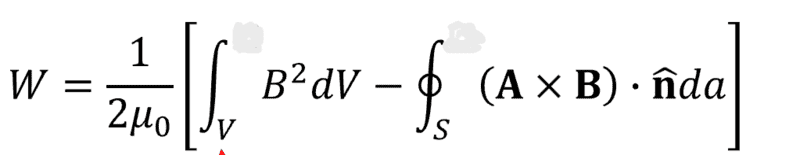# Stored magnetic energy of solenoid

• I
• Szecska8

#### Szecska8

TL;DR Summary
In my electrodynamics class there was a problem where self-inductance was calculated from the stored magnetic energy of the solenoid, which was calculated with the equation below.Can someone explain to me what the second integral represents and what is A?

Usually alphabet A is used for vector potential which generates magnetic field,
$$\nabla \times \mathbf{A}=\mathbf{B}$$

•Szecska8
The answer I got on reddit, that helped me understand it.

The total energy is given by

(1/2μ_0)∫B2dV

where the integral is taken over all space. Let us now split the space into a region X which contains the solenoid, and a region Y which is everything else. We can now write the integral as

∫B2dV=∫_X B2dV + ∫_Y B2dV

If you start from the vector calculus identity

∇•(AxB)=B•(∇xA)-A•(∇xB)

then insert the definition

∇xA=B

and Ampere’s equation

∇xB=μ_0j

which will be valid assuming static fields, you get

∇•(AxB)=B2-μ_0Aj

Outside the solenoid where there is zero current this simplifies to just

∇•(AxB)=B2

so by Gauss’ theorem we must have

∫_Y B2dV=∮_∂Y (AxB)•dS

Now the boundary ∂Y can be split into two contributions. The first is the boundary at infinity which does not contribute to the integral because the fields decay to zero sufficiently quickly. The second is the boundary shared with region X. This is exactly the same as the boundary of X with the exception that on ∂X the unit normal is oriented in the opposite direction since unit normals always point outwards. Thus

∮_∂Y (AxB)•dS=-∮_∂X (AxB)•dS

and therefore

∫B2dV=∫_X B2dV -∮_∂X (AxB)•dS

•TSny and vanhees71